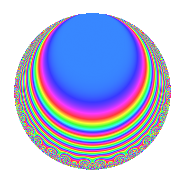# Properties

 Label 672.2.h.eLevel 672 Weight 2 Character orbit 672.h Analytic conductor 5.366 Analytic rank 0 Dimension 8 CM No Inner twists 4

# Related objects

## Newspace parameters

 Level: $$N$$ = $$672 = 2^{5} \cdot 3 \cdot 7$$ Weight: $$k$$ = $$2$$ Character orbit: $$[\chi]$$ = 672.h (of order $$2$$ and degree $$1$$)

## Newform invariants

 Self dual: No Analytic conductor: $$5.36594701583$$ Analytic rank: $$0$$ Dimension: $$8$$ Coefficient field: 8.0.40960000.1 Coefficient ring: $$\Z[a_1, \ldots, a_{7}]$$ Coefficient ring index: $$2^{4}$$ Sato-Tate group: $\mathrm{SU}(2)[C_{2}]$

## $q$-expansion

Coefficients of the $$q$$-expansion are expressed in terms of a basis $$1,\beta_1,\ldots,\beta_{7}$$ for the coefficient ring described below. We also show the integral $$q$$-expansion of the trace form.

 $$f(q)$$ $$=$$ $$q + \beta_{2} q^{3} -\beta_{4} q^{5} + \beta_{1} q^{7} + ( -2 + \beta_{6} ) q^{9} +O(q^{10})$$ $$q + \beta_{2} q^{3} -\beta_{4} q^{5} + \beta_{1} q^{7} + ( -2 + \beta_{6} ) q^{9} + 2 \beta_{3} q^{11} + ( -4 - \beta_{4} + 2 \beta_{5} ) q^{13} + ( -\beta_{1} + \beta_{3} ) q^{15} + ( -2 \beta_{4} + 2 \beta_{6} ) q^{17} + ( 4 \beta_{1} + 2 \beta_{2} + \beta_{7} ) q^{19} + ( \beta_{4} - \beta_{5} ) q^{21} -4 \beta_{7} q^{23} + 3 q^{25} + ( -\beta_{2} + 3 \beta_{7} ) q^{27} + ( 2 \beta_{4} + 2 \beta_{6} ) q^{29} + ( -4 \beta_{2} - 2 \beta_{7} ) q^{31} + ( 4 \beta_{4} + 2 \beta_{5} ) q^{33} -\beta_{7} q^{35} + ( -2 + 2 \beta_{4} - 4 \beta_{5} ) q^{37} + ( 5 \beta_{1} - 4 \beta_{2} + \beta_{3} ) q^{39} + ( -4 \beta_{4} + 2 \beta_{6} ) q^{41} + ( -2 \beta_{1} + 4 \beta_{2} + 2 \beta_{7} ) q^{43} + ( \beta_{4} + 2 \beta_{5} ) q^{45} + 4 \beta_{3} q^{47} - q^{49} + ( -2 \beta_{1} + 2 \beta_{2} + 2 \beta_{3} + 6 \beta_{7} ) q^{51} + ( -4 \beta_{4} - 2 \beta_{6} ) q^{53} + ( -4 \beta_{2} - 2 \beta_{7} ) q^{55} + ( -5 + 4 \beta_{4} - 4 \beta_{5} + \beta_{6} ) q^{57} + \beta_{7} q^{59} + ( \beta_{4} - 2 \beta_{5} ) q^{61} + ( -2 \beta_{1} - \beta_{3} ) q^{63} + ( 4 \beta_{4} - 2 \beta_{6} ) q^{65} + 2 \beta_{1} q^{67} + ( 4 + 4 \beta_{6} ) q^{69} + ( -2 \beta_{3} - 4 \beta_{7} ) q^{71} + ( -2 + 2 \beta_{4} - 4 \beta_{5} ) q^{73} + 3 \beta_{2} q^{75} + 2 \beta_{6} q^{77} + 4 \beta_{1} q^{79} + ( -1 - 4 \beta_{6} ) q^{81} + ( -4 \beta_{3} + \beta_{7} ) q^{83} + ( -4 - 2 \beta_{4} + 4 \beta_{5} ) q^{85} + ( 2 \beta_{1} + 2 \beta_{2} - 2 \beta_{3} + 6 \beta_{7} ) q^{87} + ( -4 \beta_{4} - 2 \beta_{6} ) q^{89} + ( -4 \beta_{1} + 2 \beta_{2} + \beta_{7} ) q^{91} + ( 10 - 2 \beta_{6} ) q^{93} + ( 2 \beta_{3} - 4 \beta_{7} ) q^{95} + ( 2 - 4 \beta_{4} + 8 \beta_{5} ) q^{97} + ( 10 \beta_{1} - 4 \beta_{3} ) q^{99} +O(q^{100})$$ $$\operatorname{Tr}(f)(q)$$ $$=$$ $$8q - 16q^{9} + O(q^{10})$$ $$8q - 16q^{9} - 32q^{13} + 24q^{25} - 16q^{37} - 8q^{49} - 40q^{57} + 32q^{69} - 16q^{73} - 8q^{81} - 32q^{85} + 80q^{93} + 16q^{97} + O(q^{100})$$

Basis of coefficient ring in terms of a root $$\nu$$ of $$x^{8} + 7 x^{4} + 1$$:

 $$\beta_{0}$$ $$=$$ $$1$$ $$\beta_{1}$$ $$=$$ $$($$$$\nu^{6} + 8 \nu^{2}$$$$)/3$$ $$\beta_{2}$$ $$=$$ $$($$$$\nu^{7} + \nu^{5} + 8 \nu^{3} + 8 \nu$$$$)/3$$ $$\beta_{3}$$ $$=$$ $$($$$$2 \nu^{4} + 7$$$$)/3$$ $$\beta_{4}$$ $$=$$ $$($$$$2 \nu^{7} + \nu^{5} + 13 \nu^{3} + 5 \nu$$$$)/3$$ $$\beta_{5}$$ $$=$$ $$($$$$-\nu^{7} + \nu^{5} - 8 \nu^{3} + 8 \nu$$$$)/3$$ $$\beta_{6}$$ $$=$$ $$\nu^{6} + 6 \nu^{2}$$ $$\beta_{7}$$ $$=$$ $$($$$$2 \nu^{7} - \nu^{5} + 13 \nu^{3} - 5 \nu$$$$)/3$$
 $$1$$ $$=$$ $$\beta_0$$ $$\nu$$ $$=$$ $$($$$$\beta_{7} + \beta_{5} - \beta_{4} + \beta_{2}$$$$)/2$$ $$\nu^{2}$$ $$=$$ $$($$$$-\beta_{6} + 3 \beta_{1}$$$$)/2$$ $$\nu^{3}$$ $$=$$ $$($$$$-\beta_{7} - 2 \beta_{5} - \beta_{4} + 2 \beta_{2}$$$$)/2$$ $$\nu^{4}$$ $$=$$ $$($$$$3 \beta_{3} - 7$$$$)/2$$ $$\nu^{5}$$ $$=$$ $$($$$$-8 \beta_{7} - 5 \beta_{5} + 8 \beta_{4} - 5 \beta_{2}$$$$)/2$$ $$\nu^{6}$$ $$=$$ $$4 \beta_{6} - 9 \beta_{1}$$ $$\nu^{7}$$ $$=$$ $$($$$$8 \beta_{7} + 13 \beta_{5} + 8 \beta_{4} - 13 \beta_{2}$$$$)/2$$

## Character Values

We give the values of $$\chi$$ on generators for $$\left(\mathbb{Z}/672\mathbb{Z}\right)^\times$$.

 $$n$$ $$127$$ $$421$$ $$449$$ $$577$$ $$\chi(n)$$ $$-1$$ $$1$$ $$-1$$ $$1$$

## Embeddings

For each embedding $$\iota_m$$ of the coefficient field, the values $$\iota_m(a_n)$$ are shown below.

For more information on an embedded modular form you can click on its label.

Label $$\iota_m(\nu)$$ $$a_{2}$$ $$a_{3}$$ $$a_{4}$$ $$a_{5}$$ $$a_{6}$$ $$a_{7}$$ $$a_{8}$$ $$a_{9}$$ $$a_{10}$$
575.1
 1.14412 − 1.14412i −0.437016 − 0.437016i −0.437016 + 0.437016i 1.14412 + 1.14412i −1.14412 − 1.14412i 0.437016 − 0.437016i 0.437016 + 0.437016i −1.14412 + 1.14412i
0 −0.707107 1.58114i 0 1.41421i 0 1.00000i 0 −2.00000 + 2.23607i 0
575.2 0 −0.707107 1.58114i 0 1.41421i 0 1.00000i 0 −2.00000 + 2.23607i 0
575.3 0 −0.707107 + 1.58114i 0 1.41421i 0 1.00000i 0 −2.00000 2.23607i 0
575.4 0 −0.707107 + 1.58114i 0 1.41421i 0 1.00000i 0 −2.00000 2.23607i 0
575.5 0 0.707107 1.58114i 0 1.41421i 0 1.00000i 0 −2.00000 2.23607i 0
575.6 0 0.707107 1.58114i 0 1.41421i 0 1.00000i 0 −2.00000 2.23607i 0
575.7 0 0.707107 + 1.58114i 0 1.41421i 0 1.00000i 0 −2.00000 + 2.23607i 0
575.8 0 0.707107 + 1.58114i 0 1.41421i 0 1.00000i 0 −2.00000 + 2.23607i 0
 $$n$$: e.g. 2-40 or 990-1000 Embeddings: e.g. 1-3 or 575.8 Significant digits: Format: Complex embeddings Normalized embeddings Satake parameters Satake angles

## Inner twists

Char. orbit Parity Mult. Self Twist Proved
1.a Even 1 trivial yes
3.b Odd 1 yes
4.b Odd 1 yes
12.b Even 1 yes

## Hecke kernels

This newform can be constructed as the intersection of the kernels of the following linear operators acting on $$S_{2}^{\mathrm{new}}(672, [\chi])$$:

 $$T_{5}^{2} + 2$$ $$T_{11}^{2} - 20$$